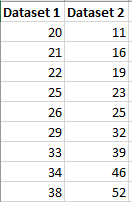# Measures of Variation: Range, IQR

A measure of variation is a summary statistic that represents the amount of dispersion in a dataset. While a measure of central tendency describes the typical value, measures of variability define how far away the data points tend to fall from the center. We talk about variability in the context of a distribution of values. A low dispersion indicates that the data points tend to be clustered tightly around the center. High dispersion signifies that they tend to fall further away.

In statistics, variability, dispersion, and spread are synonyms that denote the width of the distribution. Just as there are multiple measures of central tendency, there are several measures of variability.

### RANGE

Let’s start with the range because it is the most straightforward measure of variability to calculate and the simplest to understand. The range of a dataset is the difference between the largest and smallest values in that dataset. For example, in the two datasets below, dataset 1 has a range of 20 – 38 = 18 while dataset 2 has a range of 11 – 52 = 41. Dataset 2 has a wider range and, hence, more variability than dataset 1.While the range is easy to understand, it is based on only the two most extreme values in the dataset, which makes it very susceptible to outliers. If one of those numbers is unusually high or low, it affects the entire range even if it is atypical.

Additionally, the size of dataset affects the range. In general, you are less likely to observe extreme values. However, as you increase the sample size, you have more opportunities to obtain these extreme values. Consequently, when you draw random samples from the same population, the range tends to increase as the sample size increases. Consequently, use the range to compare variability only when the sample sizes are similar.

### THE INTERQUARTILE RANGE (IQR)

The interquartile range is the middle half of the data. To visualize it, think about the median value that splits the dataset in half. Similarly, you can divide the data into quarters. Statisticians refer to these quarters as quartiles and denote them from low to high as Q1, Q2, Q3, and Q4. The lowest quartile (Q1) contains the quarter of the dataset with the smallest values. The upper quartile (Q4) contains the quarter of the dataset with the highest values. The interquartile range is the middle half of the data that is in between the upper and lower quartiles. In other words, the interquartile range includes the 50% of data points that fall in Q2 and

The IQR is the red area in the graph below.The interquartile range is a robust measure of variability in a similar manner that the median is a robust measure of central tendency. Neither measure is influenced dramatically by outliers because they don’t depend on every value. Additionally, the interquartile range is excellent for skewed distributions, just like the median. As you’ll learn, when you have a normal distribution, the standard deviation tells you the percentage of observations that fall specific distances from the mean. However, this doesn’t work for skewed distributions, and the IQR is a great alternative.

I’ve divided the dataset below into quartiles. The interquartile range (IQR) extends from the low end of Q2 to the upper limit of Q3. For this dataset, the range is 21 – 39.## 4 thoughts on “Measures of Variation: Range, IQR”

error: Content is protected !!